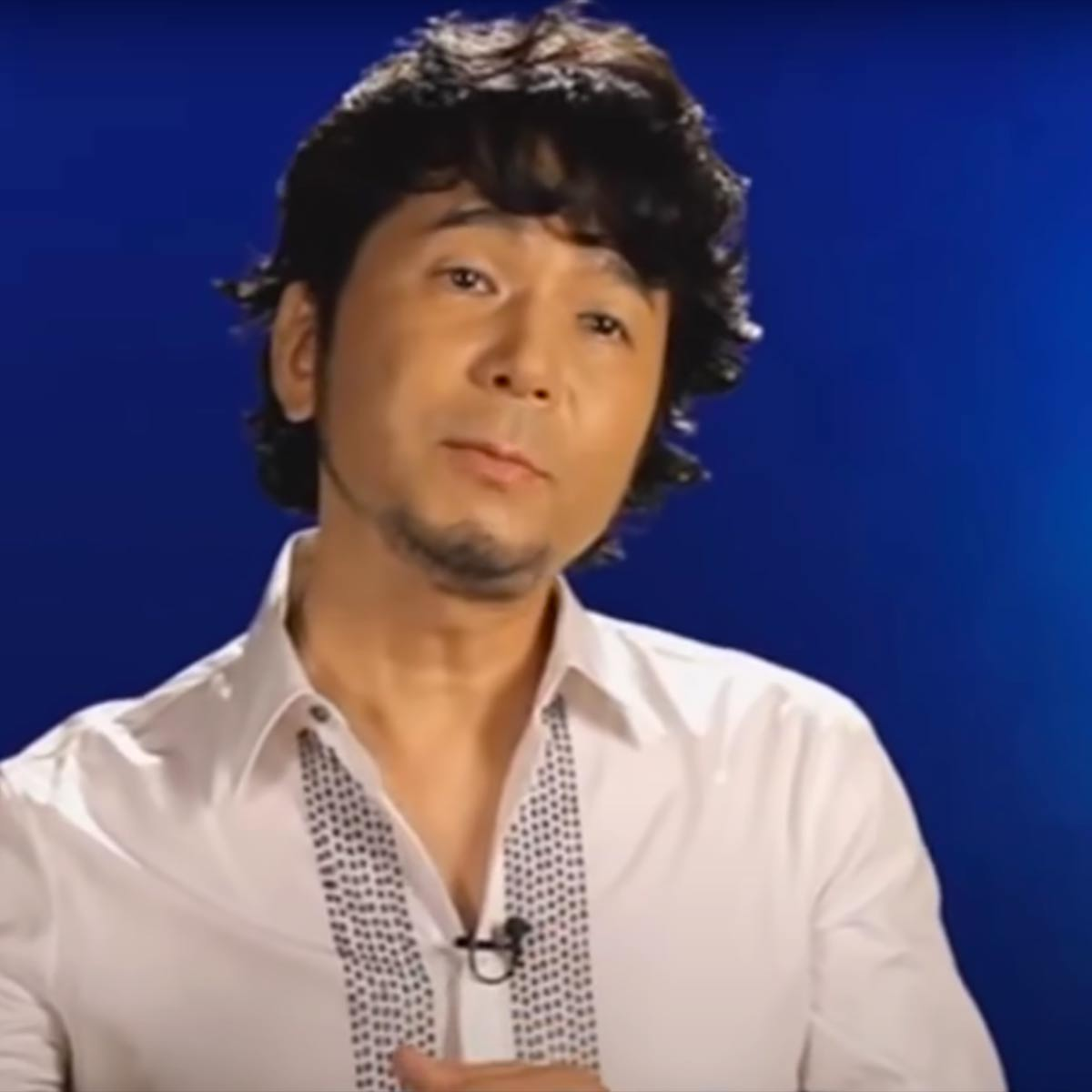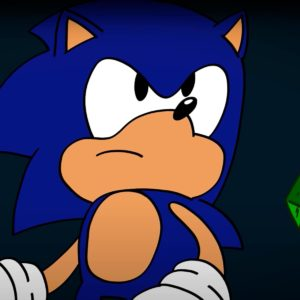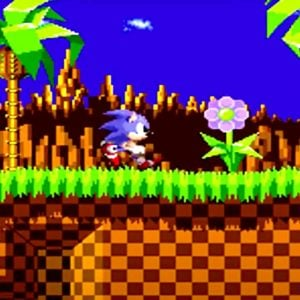# Masato Nakamura

2 Music Sheets

• ###### Birth Name:

Masato Nakamura

• ###### Born:

1st October 1958

• ###### Genres:

Masato Nakamura (Nakamura Masato) is a Japanese musician, bass guitarist, and record producer. He is a member of the J-pop band Dreams Come True, which was formed in 1988 and went on to sell over 50 million CDs. He is best known outside of Japan for composing the video game music soundtracks to Sonic the Hedgehog (1991) and Sonic the Hedgehog 2 (1992).Credit: Wikipedia

## Artist's Music Sheets

•a o a d s a p a | p I p s a p o p | o u o a p o I o I o p I y u I y | a o a d s a p a p I p s a p o p o u o a p o I o | j g j g k h k h l j l j z k z k x l x l x l x l | l j l k l k h h x z l k l k h l j l k l k h j j g j h j h s | a p a p a p s a | u o u o p a s a p o p o u p s p s d f g f d s p d s d s | e r t y a a p a a p a a p a p u t o p i p a O a a p | o p h f f g h j J j h g g f g d f g d f g h H h g f o p s s o p s s x l f g h l | h f s l J l J g J j f g h j h f h f s l J l J g J j f g h | p p a a s s S S c x z l z l j J J l J j J l b v v c c x c x z | f S S d a S S S p o a f S S d a S S S p o a h k z C C v C V b b V b | 6
Level: 3
Easy

#### Masato Nakamura

•jgjgkhkhljljzkzk [la] k j [ph] l k [ja] h l [pk] j h [la] k [pj] h [sl] k j [ha] l k [pj] h l k j h l k j h [pl] k j [ha] l k [sj] h [pl] k j [ha] l k [sj] h [sl] k j [ha] l k j h l k j h l k j h| [sf]|l [sjf]|l [kda]|l k|hjl j| [sf] j x [zsf]|l [kda]|l [kda]|hjl x| [sf]|l [sjf]|l [kda]|l k|hjl j| [sp] j j [spg]|j [oha]|j [oha]|s|| [sf]|l [sjf]|l [kda]|l k|hjl j| [sf] j x [zsf]|l [kda]|l [kda]|hjl x| [sf]|l [sjf]|l [kda]|l k|hjl j| [sp] j j [spg]|j [oha]|j [oha]|s s f [dJ]| j| h| g| f s d f p| a| s| d| f s s f [HD]| h| g| D|d d s D d| h j h|h j x| x c x v x x l|| [la] k j [ph] l k [ja] h l [pk] j h [la] k [pj] h [sl] k j [ha] l k [pj] h l k j h l k j h [pl] k j [ha] l k [sj] h [pl] k j [ha] l k [sj] h [sl] k j [ha] [la] k j h l k j h|| [xf][vh] [ml]
Level: 5
Length: 01:24
Intermediate